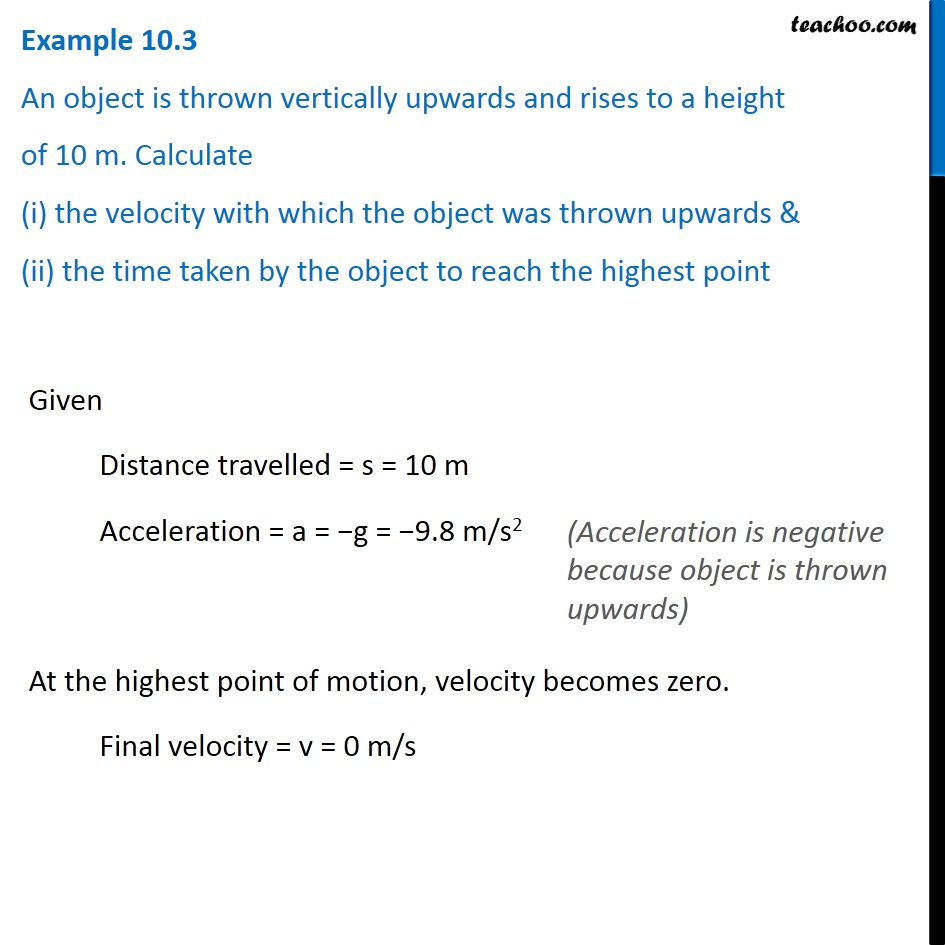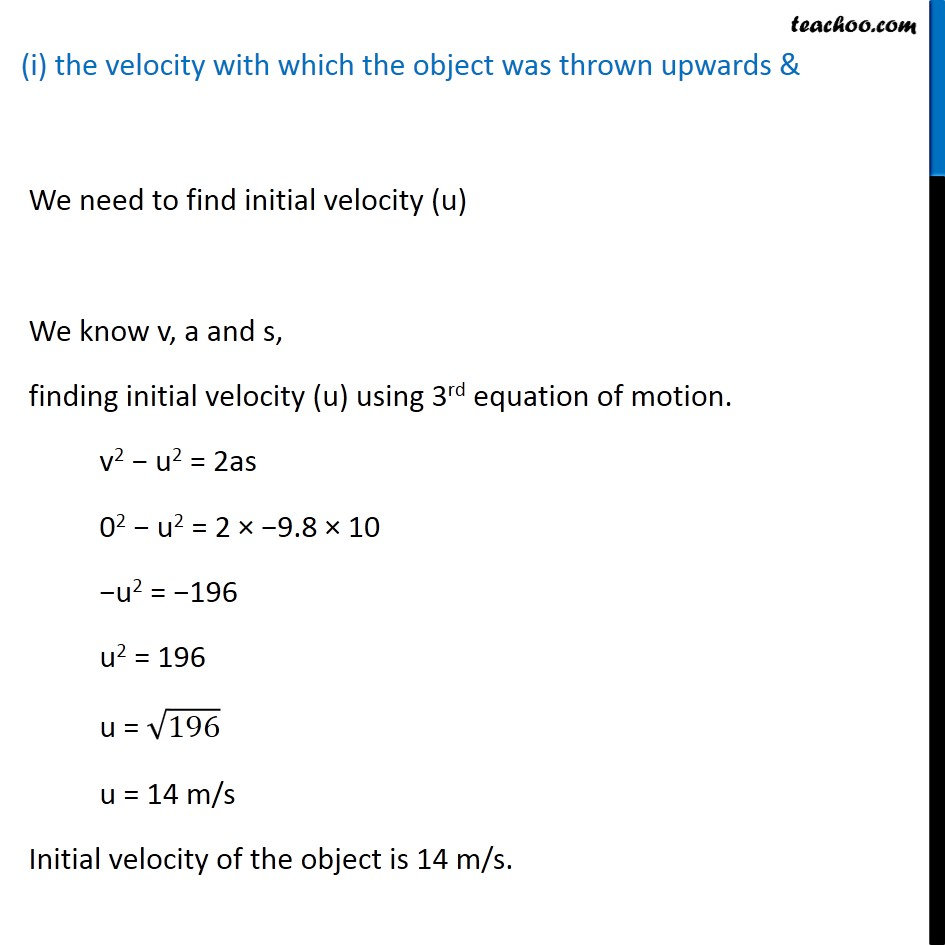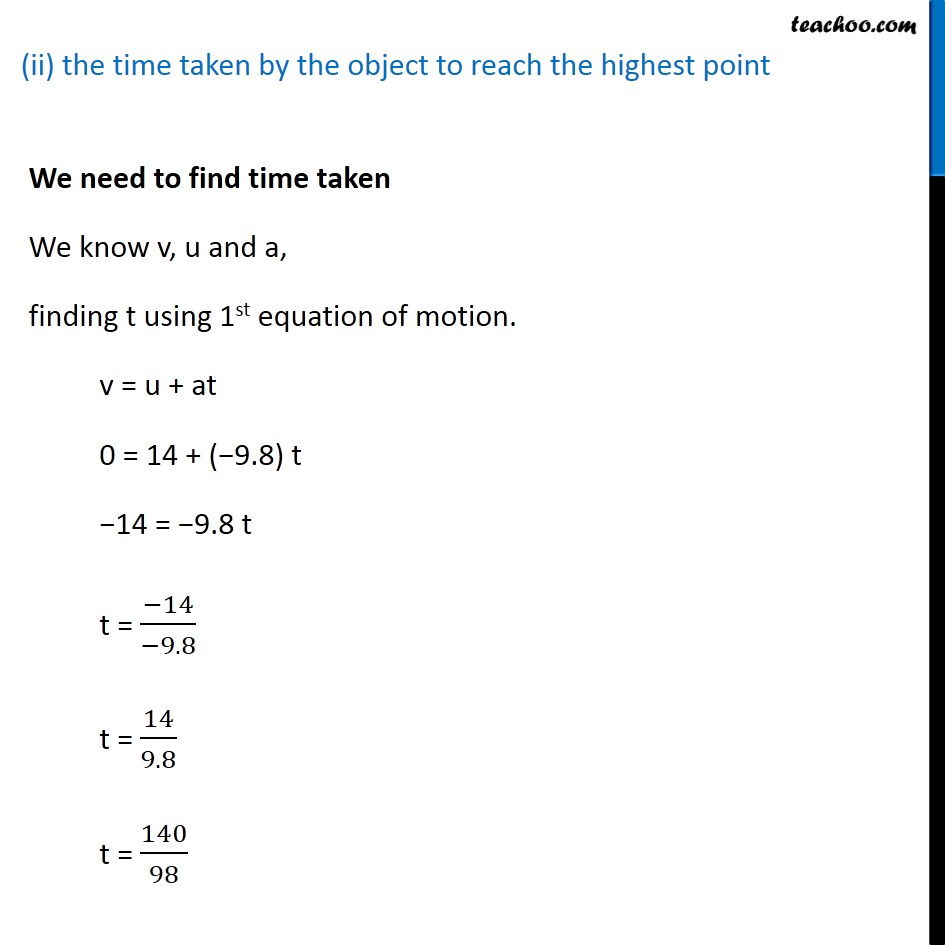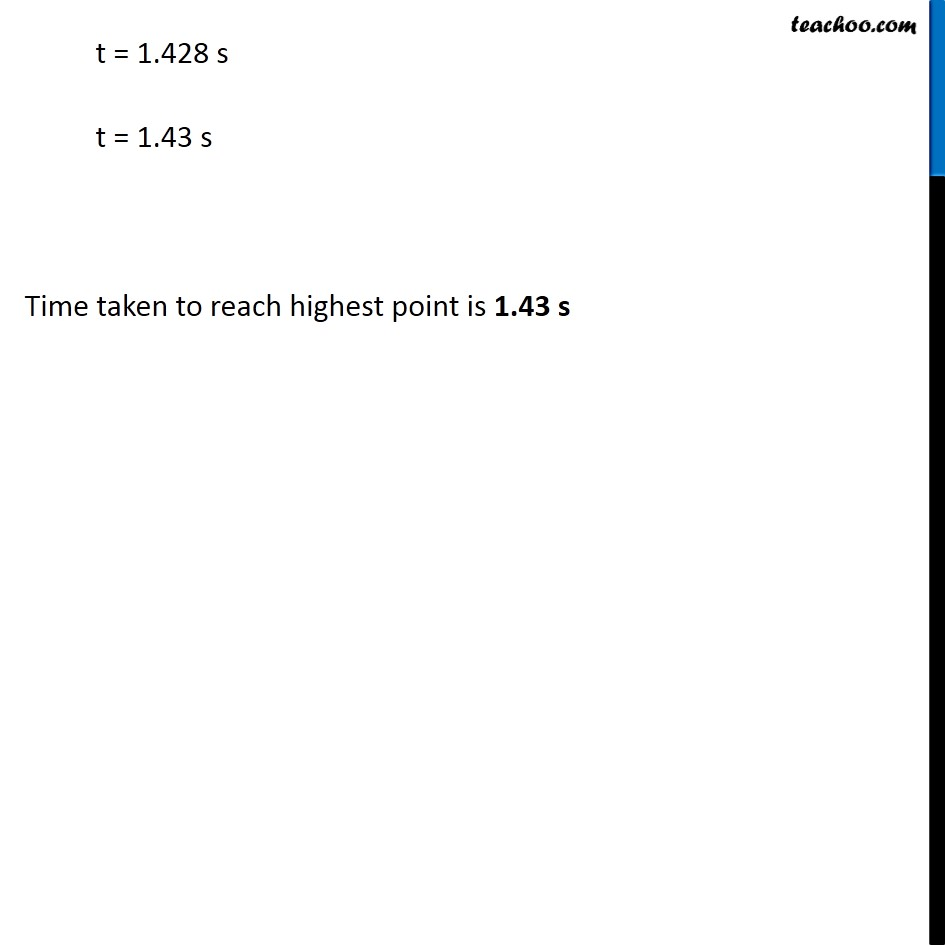Examples from NCERT Book

Class 9
Chapter 10 Class 9 - GravitationLearn in your speed, with individual attention - Teachoo Maths 1-on-1 Class

### Transcript

Example 10.3 An object is thrown vertically upwards and rises to a height of 10 m. Calculate (i) the velocity with which the object was thrown upwards & (ii) the time taken by the object to reach the highest point Given Distance travelled = s = 10 m Acceleration = a = −g = −9.8 m/s2 At the highest point of motion, velocity becomes zero. Final velocity = v = 0 m/s (Acceleration is negative because object is thrown upwards) (i) the velocity with which the object was thrown upwards & We need to find initial velocity (u) We know v, a and s, finding initial velocity (u) using 3rd equation of motion. v2 − u2 = 2as 02 − u2 = 2 × −9.8 × 10 −u2 = −196 u2 = 196 u = √196 u = 14 m/s Initial velocity of the object is 14 m/s. (ii) the time taken by the object to reach the highest point We need to find time taken We know v, u and a, finding t using 1st equation of motion. v = u + at 0 = 14 + (−9.8) t −14 = −9.8 t t = (−14)/(−9.8) t = 14/9.8 t = 140/98 t = 1.428 s t = 1.43 s Time taken to reach highest point is 1.43 s